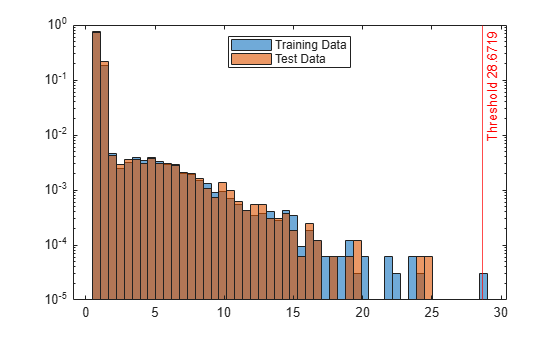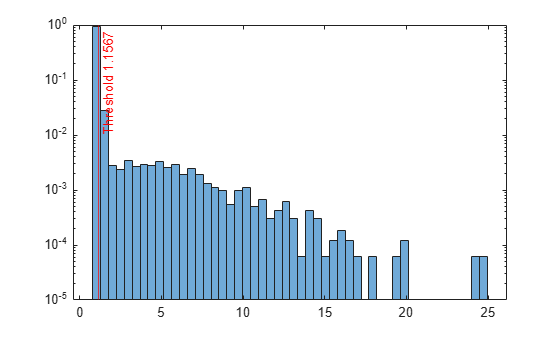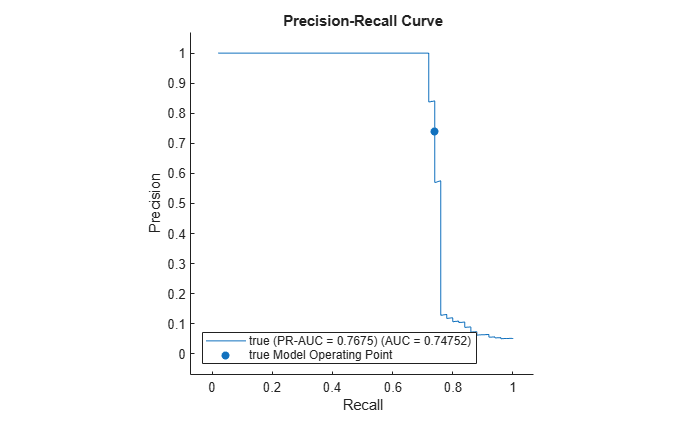# isanomaly

Find anomalies in data using local outlier factor

Since R2022b

## Syntax

``tf = isanomaly(LOFObj,Tbl)``
``tf = isanomaly(LOFObj,X)``
``tf = isanomaly(___,ScoreThreshold=scoreThreshold)``
``[tf,scores] = isanomaly(___)``

## Description

example

````tf = isanomaly(LOFObj,Tbl)` finds anomalies in the table `Tbl` using the `LocalOutlierFactor` object `LOFObj` and returns the logical array `tf`, whose elements are `true` when an anomaly is detected in the corresponding row of `Tbl`. You must use this syntax if you create `LOFObj` by passing a table to the `lof` function.```
````tf = isanomaly(LOFObj,X)` finds anomalies in the matrix `X`. You must use this syntax if you create `LOFObj` by passing a matrix to the `lof` function.```

example

````tf = isanomaly(___,ScoreThreshold=scoreThreshold)` specifies the threshold for the anomaly score, in addition to any of the input argument combinations in the previous syntaxes. `isanomaly` identifies observations with scores above `scoreThreshold` as anomalies.```
````[tf,scores] = isanomaly(___)` also returns an anomaly score, which is a local outlier factor value, for each observation in `Tbl` or `X`. A score value less than or close to 1 indicates a normal observation, and a value greater than 1 can indicate an anomaly.```

## Examples

collapse all

Create a `LocalOutlierFactor` object for uncontaminated training observations by using the `lof` function. Then detect novelties (anomalies in new data) by passing the object and the new data to the object function `isanomaly`.

Load the 1994 census data stored in `census1994.mat`. The data set consists of demographic data from the US Census Bureau to predict whether an individual makes over \$50,000 per year.

`load census1994`

`census1994` contains the training data set `adultdata` and the test data set `adulttest`. The predictor data must be either all continuous or all categorical to train a `LocalOutlierFactor` object. Remove nonnumeric variables from `adultdata` and `adulttest`.

```adultdata = adultdata(:,vartype("numeric")); adulttest = adulttest(:,vartype("numeric"));```

Train a local outlier factor model for `adultdata`. Assume that `adultdata` does not contain outliers.

`[Mdl,tf,s] = lof(adultdata);`

`Mdl` is a `LocalOutlierFactor` object. `lof` also returns the anomaly indicators `tf` and anomaly scores `s` for the training data `adultdata`. If you do not specify the `ContaminationFraction` name-value argument as a value greater than 0, then `lof` treats all training observations as normal observations, meaning all the values in `tf` are logical 0 (`false`). The function sets the score threshold to the maximum score value. Display the threshold value.

`Mdl.ScoreThreshold`
```ans = 28.6719 ```

Find anomalies in `adulttest` by using the trained local outlier factor model.

`[tf_test,s_test] = isanomaly(Mdl,adulttest);`

The `isanomaly` function returns the anomaly indicators `tf_test` and scores `s_test` for `adulttest`. By default, `isanomaly` identifies observations with scores above the threshold (`Mdl.ScoreThreshold`) as anomalies.

Create histograms for the anomaly scores `s` and `s_test`. Create a vertical line at the threshold of the anomaly scores.

```h1 = histogram(s,NumBins=50,Normalization="probability"); hold on h2 = histogram(s_test,h1.BinEdges,Normalization="probability"); xline(Mdl.ScoreThreshold,"r-",join(["Threshold" Mdl.ScoreThreshold])) h1.Parent.YScale = 'log'; h2.Parent.YScale = 'log'; legend("Training Data","Test Data",Location="north") hold off```Display the observation index of the anomalies in the test data.

`find(tf_test)`
```ans = 0x1 empty double column vector ```

The anomaly score distribution of the test data is similar to that of the training data, so `isanomaly` does not detect any anomalies in the test data with the default threshold value. You can specify a different threshold value by using the `ScoreThreshold` name-value argument. For an example, see Specify Anomaly Score Threshold.

Specify the threshold value for anomaly scores by using the `ScoreThreshold` name-value argument of `isanomaly`.

Load the 1994 census data stored in `census1994.mat`. The data set consists of demographic data from the US Census Bureau to predict whether an individual makes over \$50,000 per year.

`load census1994`

`census1994` contains the training data set `adultdata` and the test data set `adulttest`.

Remove nonnumeric variables from `adultdata` and `adulttest`.

```adultdata = adultdata(:,vartype("numeric")); adulttest = adulttest(:,vartype("numeric"));```

Train a local outlier factor model for `adultdata`.

`[Mdl,tf,scores] = lof(adultdata);`

Plot a histogram of the score values. Create a vertical line at the default score threshold.

```h = histogram(scores,NumBins=50,Normalization="probability"); h.Parent.YScale = 'log'; xline(Mdl.ScoreThreshold,"r-",join(["Threshold" Mdl.ScoreThreshold]))```Find the anomalies in the test data using the trained local outlier factor model. Use a different threshold from the default threshold value obtained when training the local outlier factor model.

First, determine the score threshold by using the `isoutlier` function.

`[~,~,U] = isoutlier(scores)`
```U = 1.1567 ```

Specify the value of the `ScoreThreshold` name-value argument as `U`.

```[tf_test,scores_test] = isanomaly(Mdl,adulttest,ScoreThreshold=U); h = histogram(scores_test,NumBins=50,Normalization="probability"); h.Parent.YScale = 'log'; xline(U,"r-",join(["Threshold" U]))```Generate a sample data set that contains outliers. Compute anomaly scores for the points around the sample data by using the `isanomaly` function, and create a contour plot of the anomaly scores. Then, check the performance of the trained local outlier model by plotting the precision-recall curve.

Use a Gaussian copula to generate random data points from a bivariate distribution.

```rng("default") rho = [1,0.05;0.05,1]; n = 1000; u = copularnd("Gaussian",rho,n);```

Add noise to 5% of randomly selected observations to make the observations outliers.

```noise = randperm(n,0.05*n); true_tf = false(n,1); true_tf(noise) = true; u(true_tf,1) = u(true_tf,1)*5;```

Train a local outlier factor model by using the `lof` function. Set the fraction of anomalies in the training observations to 0.05. For better performance, you can also modify the local outlier factor algorithm options by specifying name-value arguments, such as `SearchMethod`, `NumNeighbors`, and `Distance`. In this case, specify the number of nearest neighbors to use as 40.

`[LOFObj,tf,scores] = lof(u,ContaminationFraction=0.05,NumNeighbors=40);`

Compute anomaly scores for 2-D grid coordinates around the training observations by using the trained local outlier factor model and the `isanomaly` function.

```l1 = linspace(min(u(:,1),[],1),max(u(:,1),[],1)); l2 = linspace(min(u(:,2),[],1),max(u(:,2),[],1)); [X1,X2] = meshgrid(l1,l2); [~,scores_grid] = isanomaly(LOFObj,[X1(:),X2(:)]); scores_grid = reshape(scores_grid,size(X1,1),size(X2,2));```

Create a scatter plot of the training observations and a contour plot of the anomaly scores. Flag true outliers and the outliers detected by `lof`.

```idx = setdiff(1:1000,noise); scatter(u(idx,1),u(idx,2),[],[0.5 0.5 0.5],".") hold on scatter(u(noise,1),u(noise,2),"ro","filled") scatter(u(tf,1),u(tf,2),60,"kx",LineWidth=1) contour(X1,X2,scores_grid,"ShowText","on") legend(["Normal Points" "Outliers" "Detected Outliers"],Location="best") colorbar hold off```Check the performance of the trained local outlier factor model by plotting the precision-recall curve and computing the area under the curve (AUC) value. Create a `rocmetrics` object. `rocmetrics` computes the false positive rates and the true positive rates (or recall) by default. Specify the `AdditionalMetrics` name-value argument to additionally compute the precision values (or positive predictive values).

`rocObj = rocmetrics(true_tf,scores,true,AdditionalMetrics="PositivePredictiveValue");`

Plot the curve by using the `plot` function of `rocmetrics`. Specify the y-axis metric as precision (or positive predictive value) and the x-axis metric as recall (or true positive rate). Display a filled circle at the model operating point corresponding to `LOFObj.ScoreThreshold`. Compute the area under the precision-recall curve using the trapezoidal method of the `trapz` function, and display the value in the legend.

```r = plot(rocObj,YAxisMetric="PositivePredictiveValue",XAxisMetric="TruePositiveRate"); hold on idx = find(rocObj.Metrics.Threshold>=LOFObj.ScoreThreshold,1,'last'); scatter(rocObj.Metrics.TruePositiveRate(idx), ... rocObj.Metrics.PositivePredictiveValue(idx), ... [],r.Color,"filled") xyData = rmmissing([r.XData r.YData]); auc = trapz(xyData(:,1),xyData(:,2)); legend(join([r.DisplayName " (AUC = " string(auc) ")"],""),"true Model Operating Point") xlabel("Recall") ylabel("Precision") title("Precision-Recall Curve") hold off```## Input Arguments

collapse all

Trained local outlier factor model, specified as a `LocalOutlierFactor` object.

Predictor data, specified as a table. Each row of `Tbl` corresponds to one observation, and each column corresponds to one predictor variable. Multicolumn variables and cell arrays other than cell arrays of character vectors are not allowed.

If you train `LOFObj` using a table, then you must provide predictor data by using `Tbl`, not `X`. All predictor variables in `Tbl` must have the same variable names and data types as those in the training data. However, the column order in `Tbl` does not need to correspond to the column order of the training data.

Data Types: `table`

Predictor data, specified as a numeric matrix. Each row of `X` corresponds to one observation, and each column corresponds to one predictor variable.

If you train `LOFObj` using a matrix, then you must provide predictor data by using `X`, not `Tbl`. The variables that make up the columns of `X` must have the same order as the training data.

Data Types: `single` | `double`

Threshold for the anomaly score, specified as a nonnegative scalar. `isanomaly` identifies observations with scores above the threshold as anomalies.

The default value is the `ScoreThreshold` property value of `LOFObj`.

Example: `ScoreThreshold=0.5`

Data Types: `single` | `double`

## Output Arguments

collapse all

Anomaly indicators, returned as a logical column vector. An element of `tf` is `true` when the observation in the corresponding row of `Tbl` or `X` is an anomaly, and `false` otherwise. `tf` has the same length as `Tbl` or `X`.

`isanomaly` identifies observations with `scores` above the threshold (the `ScoreThreshold` value) as anomalies.

Anomaly scores (local outlier factor values), returned as a numeric column vector whose values are nonnegative. `scores` has the same length as `Tbl` or `X`, and each element of `scores` contains an anomaly score for the observation in the corresponding row of `Tbl` or `X`. A score value less than or close to 1 can indicate a normal observation, and a value greater than 1 can indicate an anomaly.

collapse all

### Local Outlier Factor

The local outlier factor (LOF) algorithm detects anomalies based on the relative density of an observation with respect to the surrounding neighborhood.

The algorithm finds the k-nearest neighbors of an observation and computes the local reachability densities for the observation and its neighbors. The local outlier factor is the average density ratio of the observation to its neighbor. That is, the local outlier factor of observation p is

`$LO{F}_{k}\left(p\right)=\frac{1}{|{N}_{k}\left(p\right)|}\sum _{o\in {N}_{k}\left(p\right)}\frac{lr{d}_{k}\left(o\right)}{lr{d}_{k}\left(p\right)},$`

where

• lrdk(·) is the local reachability density of an observation.

• Nk(p) represents the k-nearest neighbors of observation p. You can specify the `IncludeTies` name-value argument as `true` to include all the neighbors whose distance values are equal to the kth smallest distance, or specify `false` to include exactly k neighbors. The default `IncludeTies` value of `lof` is `false` for more efficient performance. Note that the algorithm in  uses all the neighbors.

• |Nk(p)| is the number of observations in Nk(p).

For normal observations, the local outlier factor values are less than or close to 1, indicating that the local reachability density of an observation is higher than or similar to its neighbors. A local outlier factor value greater than 1 can indicate an anomaly. The `ContaminationFraction` argument of `lof` and the `ScoreThreshold` argument of `isanomaly` control the threshold for the local outlier factor values.

The algorithm measures the density based on the reachability distance. The reachability distance of observation p with respect to observation o is defined as

`${\stackrel{˜}{d}}_{k}\left(p,o\right)=\mathrm{max}\left({d}_{k}\left(o\right),d\left(p,o\right)\right),$`

where

• dk(o) is the kth smallest distance among the distances from observation o to its neighbors.

• d(p,o) is the distance between observation p and observation o.

The algorithm uses the reachability distance to reduce the statistical fluctuations of d(p,o) for the observations close to observation o.

The local reachability density of observation p is the reciprocal of the average reachability distance from observation p to its neighbors.

`$lr{d}_{k}\left(p\right)=1/\frac{\sum _{o\in {N}_{k}\left(p\right)}{\stackrel{˜}{d}}_{k}\left(p,o\right)}{|{N}_{k}\left(p\right)|}.$`

The density value can be infinity if the number of duplicates is greater than the number of neighbors (k). Therefore, if the training data contains duplicates, the `lof` and `isanomaly` functions use the weighted local outlier factor (WLOF) algorithm. This algorithm computes the weighted local outlier factors using the weighted local reachability density (wlrd).

`$WLO{F}_{k}\left(p\right)=\frac{1}{\sum _{o\in {N}_{k}\left(p\right)}w\left(o\right)}\sum _{o\in {N}_{k}\left(p\right)}\frac{wlr{d}_{k}\left(o\right)}{wlr{d}_{k}\left(p\right)},$`

where

`$wlr{d}_{k}\left(p\right)=1/\frac{\sum _{o\in {N}_{k}\left(p\right)}w\left(o\right){\stackrel{˜}{d}}_{k}\left(p,o\right)}{\sum _{o\in {N}_{k}\left(p\right)}w\left(o\right)},$`

and w(o) is the number of duplicates for observation o in the training data. After computing the weight values, the algorithm treats each set of duplicates as one observation.

## Algorithms

• To compute the local outlier factor values (`scores`) for each observation in `Tbl` or `X`, `isanomaly` finds the k-nearest neighbors among the training observations stored in the `X` property of a `LocalOutlierFactor` object.

• `isanomaly` considers `NaN`, `''` (empty character vector), `""` (empty string), `<missing>`, and `<undefined>` values in `Tbl` and `NaN` values in `X` to be missing values.

• `isanomaly` does not use observations with missing values.

• `isanomaly` assigns the anomaly score of `NaN` and anomaly indicator of `false` (logical 0) to observations with missing values.

 Breunig, Markus M., et al. “LOF: Identifying Density-Based Local Outliers.” Proceedings of the 2000 ACM SIGMOD International Conference on Management of Data, 2000, pp. 93–104.